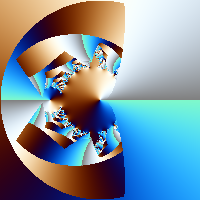## Regions

All Fract-O-Rama formula files have a section that looks like this:

```  formula
{
zero or more statements

while(some_condition)
{
zero or more statements
}

zero or more statements
}
```

The 'while(some_condition)' part specifies the conditions under which the program will continue to execute the statements inside the while loop.

Here is a complete formula file with a typical while loop condition:

```  fractal
{
mapping { (-2, -2, 2, 2) => (200, 200) }

formula
{
z = [0, 0];

while(\$count < 20 && ssq(z) < 4)
{
z = z ^ 2 + current;
}

\$value = deg(z) * (255 / 360.0);

set_color(\$value, \$value, \$value);
}
}
```

Many interesting fractals can be generated by varying the condition that governs the while loop. Regions are one such mechanism, they provide a way to test to see if a point is within a certain area. Typically this is used in a manner like this:

```  fractal
{
mapping { (-2, -2, 2, 2) => (200, 200) }

formula
{
z = [0, 0];

while(\$count < 20 && ssq(z) < 4)
{
if(inside(z, /* some region */))
{
// our 'z' point is inside the region, leave the
// while loop prematurely

break;
}
z = z ^ 2 + current;
}

\$value = deg(z) * (255 / 360.0);

set_color(\$value, \$value, \$value);
}
}
```
Above /* some region */ is a placeholder for an actual region but we can see how we go about testing to see if a point is within a region and now we'll look at the different types of regions that are available.

There are 6 types of regions and 4 ways to combine regions. First, we'll look at the region types.

Region Explanation
`r_circle(center, \$radius)`
`r_cross(p1, p2, \$barWidth, \$barHeight)`
This region describes a rectangular area (p1 and p2 are the corners). The cross is incribed within the rectangular region and the thickness of the horizontal bar is '\$barHeight' the thickness of the vertical bar is '\$barWidth'
`r_ellipse(center, \$xradius, \$yradius)`
This region describes an ellipse whose center is 'center'. The width of the ellipse is governed by '\$xradius' its height by '\$yradius'
`r_poly(p1, p2, ..., pN)`
This region is a polygon defined by the points p1, p2, ..., pN. There must be at least 3 points but there is limit on the maximum number of points
`r_spoly(center, \$nSides, \$radius, \$angle)`
This region describes an '\$nSides'-sided polygon whose center is 'center'. The value '\$radius' indicates the distance from the center to any of the polygon's '\$nSides' points. The '\$angle' value rotates the polygon around its center, if this value is zero one point of the polygon will be at 0 degrees (3 o'clock).
`r_rect(p1, p2)`
This region describes a rectangle whose opposite corners are the points 'p1' and 'p2'

Now let's look at some images of what these regions actually look like. For this discussion we'll assume the following:

• Our drawing area is a square located at (0, 0), (1, 1)
• All our regions will be specified relative to our drawing area's coordinates`r_circle([.5, 5.], .4)``r_circle([.4, 4.], .2)``r_cross([.2, .2], [.8, .8], .2, .4)``r_cross([.1, .1], [.9, .9], .3, .1)``r_ellipse([.5, .5], .4, .2)`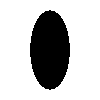`r_ellipse([.5, .5], .2, .4)````r_poly([.2, 0], [.3, .3], [.2, .3], [.3, .6],
[.2, .6], [.4, 1],  [.3, .7],[.4, .7],
[.3, .4],[.4, .4], [.2, 0])```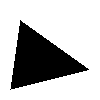`r_poly([.1, .1], [.2, .8], [.9, .3])``r_spoly([.5, .5], 5, .4, 0)``r_spoly([.5, .5], 6, .4, 20)``r_rect([.1, .1], [.7, .8])``r_rect([.3, .4], [.9, .1])`

Now lets look at the mechanisms for combining regions

 r_and(region1, region2) Specifies the area that is in both region1 and region2 r_or(region1, region2) Specifies the area that is in either region1 or region2 r_xor(region1, region2) Specifies the area that is in either region1 or region2 but not both r_not(region) Specifies the area that is not in region

For these examples, we'll be using the following regions/images:`region1: r_circle([.3, 5.], .25)``region2: r_circle([.6, 5.], .25)`

Here are images showing the different mechanisms for combining regions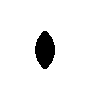`r_and(region1, region2)`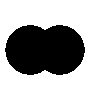`r_or(region1, region2)`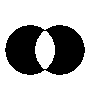`r_xor(region1, region2)``r_not(region1)`

Finally, it is worth noting that the combining mechanisms (r_and, r_or, r_xor, r_not) are regions themselves so they can also be combined. Here are some examples: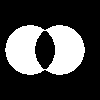`r_not(r_xor(region1, region2))``r_not(r_or(region1, region2))`

And here is a complete formula file that uses regions and the image it produces

```   fractal
{
mapping {
(-2.00000000000000000000,
-2.00000000000000000000,
2.00000000000000000000,
2.00000000000000000000) => (200, 200)
}

formula
{
z  = [0, 0];
p  = [1, 0];
\$n = 3;
\$r = 2.0;
\$a = 0.0;

while(\$count < 20 && ssq(z) < 4)
{
z = z ^ 2 + current;

z1 = asech(z);
z2 = conj(z1);

if(
inside(z1, r_spoly(p, \$n, \$r, \$a)) ||
inside(z2, r_spoly(p, \$n, \$r, \$a))
)
{
break;
}

}

\$r = \$g = \$b = 0;

\$value = deg(z) / 360.0 * 2.0 * \$m_pi;

\$r     = 127.5 + 127.5 * sin(1.0 * \$value);
\$g     = 127.5 + 127.5 * sin(1.2 * \$value);
\$b     = 127.5 + 127.5 * sin(1.4 * \$value);

set_color(\$r, \$g, \$b);
}
}
```